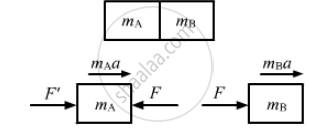# P Two Blocks a and B of Mass Ma and Mb , Respectively, Are Kept in Contact on a Frictionless Table. the Experimenter Pushes Block a from - Physics

Sum

Two blocks A and B of mass mA and mB , respectively, are kept in contact on a frictionless table. The experimenter pushes block A from behind, so that the blocks accelerate. If block A exerts force F on block B, what is the force exerted by the experimenter on block A?

#### Solution

Let F' = force exerted by the experimenter on block A and F be the force exerted by block A on block B.Let a be the acceleration produced in the system.
For block A,
$F' - F = m_A a$   ...(1)
For block B,
F = mBa    ...(2)
Dividing equation (1) by (2), we get:
$\frac{F'}{F} - 1 = \frac{m_A}{m_B}$
$\Rightarrow F' = F\left( 1 + \frac{m_A}{m_B} \right)$
∴ Force exerted by the experimenter on block A is
$F\left( 1 + \frac{m_A}{m_B} \right)$

Concept: Newton’s Second Law of Motion
Is there an error in this question or solution?

#### APPEARS IN

HC Verma Class 11, Class 12 Concepts of Physics Vol. 1
Chapter 5 Newton's Laws of Motion
Q 7 | Page 79